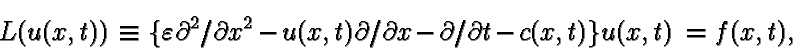Discrete Approximation of the Riemann Problem for the Viscous Burgers Equation

In this paper we consider discrete approximations of a Dirichlet problem for the quasilinear parabolic equationthat is, the viscous Burgers equation. The singular perturbation parameter e takes arbitrary values from the half-interval (0,1]. The initial condition has a discontinuity of the first kind at the point S*=(0,0) such that j0(+0)-j0(-0)>0, where u(x,0)=j0(x); thus, we have the Riemann problem. For such a problem we construct special finite difference schemes controlled by the parameter e and by the type of the singularities, which the solution u(x,t) exhibits. The discrete solution for this problem is shown to converge uniformly with respect to the parameter e in a uniform grid metric.